# 考虑破损-安全的连续体结构拓扑优化ICM方法

1 湖南城市学院土木工程学院,湖南益阳 413000
2 北京工业大学工程数值模拟中心,北京 100022

# ICM METHOD FOR FAIL-SAFE TOPOLOGY OPTIMIZATION OF CONTINUUM STRUCTURES

1 School of Civil Engineering, Hunan City University, Yiyang 413000, Hunan, China
2 Numerical Simulation Center for Engineering, Beijing University of Technology, Beijing 100022, China

Abstract

Aimed at the topology optimization of continuum structures considering the fail-safe principle, for the purpose of overcoming the shortcomings of the topologies obtained by the traditional topology optimization being too sensitive to local damages for the lack of reasonable redundancy components, the fail-safe design is achieved. At first, four concepts are clarified: the structural local failure mode, the structural local failure region, structural failure case, and the pre-estimation distribution of structural failure cases. Secondly, based on the ICM (independent continuous mapping) method, a minimizing structural volume model with structural performance constraints is established for the fail-safe topology optimization problems of continuum structures. While establishing the objective function, minimizing the maximum of structural volumes of all structural failure cases is converted into minimizing the structural volume of the ground structure without failure regions. Therefore, the difficulty of dealing with multi-objective optimization is avoided. While establishing the approximation functions of constraints, mechanical property constraints of all of the structural failure cases are taken into account. The problems with a single load case or multi-load cases can be solved by the presented model. At last, optimization problems with displacement constraints are taken as examples. The optimization model is established and the solution method is also presented. Some examples with displacement constraints under a single load case or multi-load case are presented to verify the validity of this method. The results show that the optimal topologies obtained by this method are more complex and has a greater volume than that obtained by the topology optimization without fail-safe. Namely, optimal topologies have more redundancy, which is the result of considering the fail-safe principle. The proposed research is an important progress for the design of vehicles serving for aviation, aerospace, water or land fields and other engineering structures undergoing accident damages, war wounds or terrorist attacks.

Keywords： continuum structures ; topology optimization ; fail-safe ; ICM method

0

Peng Xirong, Sui Yunkang. ICM METHOD FOR FAIL-SAFE TOPOLOGY OPTIMIZATION OF CONTINUUM STRUCTURES[J]. Chinese Journal of Theoretical and Applied Mechanics, 2018, 50(3): 611-621 https://doi.org/10.6052/0459-1879-17-366

## 引言

Sun和Arora[7,8]于1976年提出破损-安全结构优化设计,在桁架结构杆件截面优化设计中考虑结构局 部破坏的影响. Nguyen和Arora对复合结构进行破损-安全结构优化设计. 由于局部破坏的位置与形式的不确定性,如何考虑结构的局部破坏成为破损-安全结构优化的研究难点. 在Arora等[7,8,9]的工作中,通过预先指定结构破损模式,将结构破损-安全优化问题归结为考虑各种 结构破损状况下 性能约 束的传统结构优化问题. Feng等[10,11]针对截面优化问题探讨了结构构型与冗余间关系. Marhadi等 [12,13]考虑非线性分析,研究了局部破损对桁架结构最优设计结果的影响. 杜剑明等提出桁架结构破损-安全优化的双层规划法,上层规划寻求杆件的最优尺寸,下层优化确定结构 最不利 破损状况,避免了人为设定破损状况的不足. Bendsøe等[15,16]研究了材料性能退化对桁架及连续体结构拓扑优化的影响.

Zhou等为了克服上述困难,选取适当大小的局部破损区域,与邻近的局部破损区域中心距离拉开,使得含局部破损区域的 基机构数目骤然下降,其对应的柔顺度数量也极大减小,令相应的建模与求解的计算能够被接受,可贵的是,文献对采取的做 法进行了理论论证. 这是Zhou等做出的重大贡献.

(1)系统阐述了破损-安全拓扑优化的基本概念,如结构局部破损模式(structural local failure mode)、结构局部破损区域(structural local failure region)、结构破损状况(structural failure case)、结构破损状况的预估分布(pre-estimation distribution of structural failure cases)等.

(2)代替目前破损-安全拓扑优化研究中以结构柔顺度为目标函数,转换为含结构局部破损区域的全部基结构的最大体积的极小化为目标,避免结构柔顺度为优化目标建模时需要先验地确定结构体积的不合理性.

(3)基于Minimax的概念,将全部结构破损状况的最大体积的极小化的问题转化为单个的目标,避免结构柔顺度的优化目标模型需要利用应用K-S函数方法凝聚目标进行求解的繁重计算量.

(4)基于上述策略,依托结构拓扑优化ICM方法,建立了结构力学性能约束下体积极小化的破损-安全拓扑优化模型, 不仅避免了以往研究先验地指定体积比约束的不合理性,而且对多载荷工况、多性能约束问题,都能够有效地建模.

(5)采用对偶序列二次规划法求解优化模型.

## 1 连续体结构破损-安全拓扑优化建模前处理

### 的基本概念和策略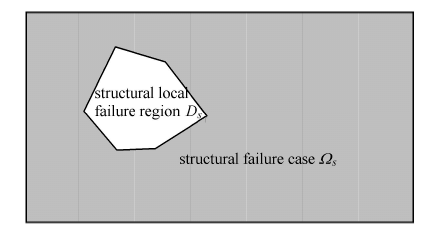Fig. 1   Structural local failure region and structural failure case

### 1.4 结构破损状况的预估分布

$Φ=Ωs|Ωs=Ω-Ds(s=1,2,⋯,S)(1)$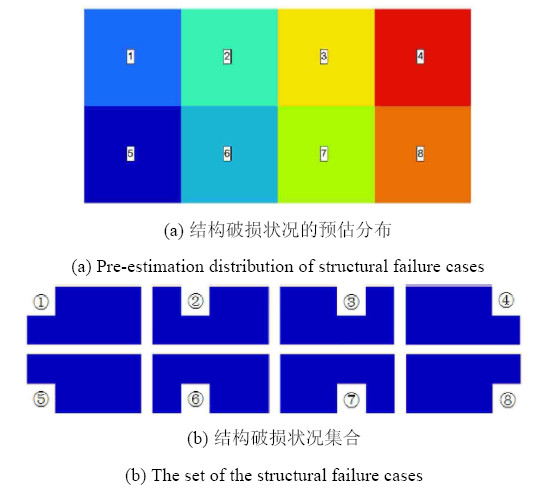Fig. 2   An example of fail-safe design while structural local failure mode taking square

## 2 连续体结构破损-安全拓扑优化模型

### 2.1 一般表达和基于结构破损状况的模型

$\left.\begin{array}{ll} {\rm Find } \ & {\pmb x} \in E^N \\ {\rm Make } \ & f ( x,\varPhi ) \to {\rm min} \\ {\rm s.t.} \ \ & g_j (x,\varPhi ) \leqslant \overline g_j , j = 1,2, \cdots ,J \\ & 0 \leqslant x_i \leqslant 1 , \ \ i = 1,2, \cdots ,N \end{array} \right\} (2)$

### 2.2 目标函数为结构柔顺度的模型

Jansen等及Zhou等之所以能对式(2)进行求解,是因为其对结构破损状况进行了预估分布,他们研究的均是体积约束下柔顺度极小的拓扑优化问题,其拓扑模型为

$\left.\begin{array}{ll} {\rm Find } \ & {\pmb x} \in E^N \\ {\rm Make } \ & \mathop {\max}\limits_{s = 1,2,\cdots,S} C_s (x) \to \min \\ {\rm s.t.} \ & V (x) \leqslant \overline V \\ & 0 \leqslant x_i \leqslant 1 ; \ i = 1,2, \cdots ,N \end{array} \right \} (3)$

### 2.3 基于ICM方法目标函数为结构体积的模型

$\left.\begin{array}{ll} {\rm Find } & {\pmb t} \in E^N \\ {\rm Make } & V(\pmb t,\varPhi) = \mathop {\max}\limits_{s = 1,2,\cdots,S} V_s ( t) \to \min \\ {\rm s.t.} & u_{jls} ({\pmb t}) \leqslant \overline {u_j } \\ & (j = 1,2,\cdots,J ; \ l = 1,2,\cdots,L ; & \ s = 1,2,\cdots,S) \\ & \underline{t_i } \leqslant t_i \leqslant 1 \ (i = 1,2, \cdots ,N) \end{array}\!\! \right \} (4)$

$V_s ({\pmb t}) = V({\pmb t}) - v_s ({\pmb t}) (5{\rm a})$

$V_s ({\pmb t}) \leqslant V({\pmb t}) (5{\rm b})$

$\mathop {\max}\limits_{s = 1,2,\cdots,S} V_s ({\pmb t}) \leqslant V({\pmb t}) (6)$

$\left.\begin{array}{ll} {\rm Find } & {\pmb t} \in E^N \\ {\rm Make } & V({\pmb t}) = \sum_{i = 1}^N f_v (t_i )v_i^0 \to \min \\ {\rm s.t.} & u_{jls} ({\pmb t}) \leqslant \overline {u_j } \\ & ( j = 1,2,\cdots,J ; \ l = 1,2,\cdots,L ; \ s = 1,2, \cdots,S) \\ & \underline{t_i } \leqslant t_i \leqslant 1 \ (i = 1,2,\cdots ,N) \end{array} \right \} (7)$

### 2.4 基于ICM方法的约束显式化及模型的求解

2.3节以某个预估排列方式布置结构局部破损区域,得到所有结构破损状况对应的基结构 $fk(ti)$,连同载荷工况和多个位移约束一并考虑到建模之中,建立了式(7)表述的优化模型.

$x_i = \dfrac{1}{f_k (t_i )} = \dfrac{1}{t_i^\alpha } (8)$

$u_m = \sum_{i = 1}^N \dfrac{x_i }{x_i^{(k)} } ({\pmb\delta}_{im}^{V})^{\rm T} {\pmb F}_{im}^{R} (9)$

$A_{im} = ({\pmb\delta}_{im}^{\rm V} )^{\rm T}{\pmb F}_{im}^{\rm R} (10)$

$c_{im} = A_{im} / x_i^{( k)} (11)$

$u_m = \sum_{i = 1}^N \dfrac{x_i }{x_i^{(k)} } ({\pmb\delta}_{im}^{\rm V} )^{\rm T}{\pmb F}_{im}^{\rm R} =\sum_{i = 1}^N c_{im} x_i (12)$

$\left.\begin{array}{ll} {\rm Find } & {\pmb x} \in E^N \\ {\rm Make } & V({\pmb x}) = \sum_{i = 1}^N v_i^0 / x_i^\alpha \to \min \\ {\rm s.t.} & u_m ({\pmb x}) = \sum_{i = 1}^N c_{im} x_i \leqslant \overline {u_m } \\ & m = 1,2, \cdots ,M \\ & 1 \leqslant x_i \leqslant \overline {x_i } ; \ i = 1,2, \cdots ,N \end{array}\!\! \right \} (13)$

$\varDelta = \mathop {\max}\limits_{i = 1,2,\cdots,N} ( \vert t_i^{(k + 1)} - t_i^{(k)} \vert ) \leqslant \varepsilon (14)$

## 3 数值算例及讨论

### 3.1 算例1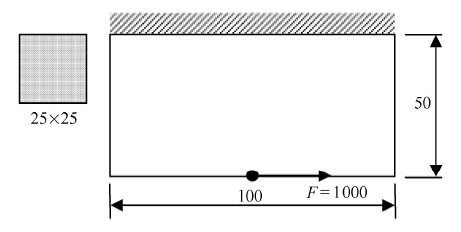Fig. 3   Definition of the topology design problem for example 1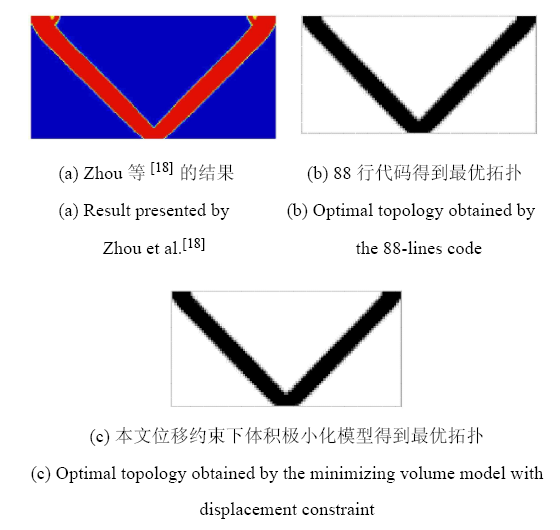Fig. 4   Optimal topologies obtained by topology optimization without fail-safe

Zhou等 得到的考虑破损-安全的最优拓扑如图5(a)所示,其体积约束仍为20%,在相同体积约束下,与不考虑破损-安全的拓扑优化相比,结构更为复杂.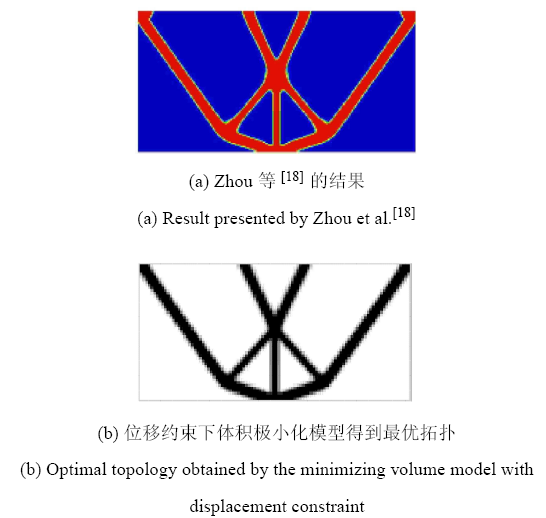Fig. 5   Optimal topologies obtained by the topology optimization with fail-safe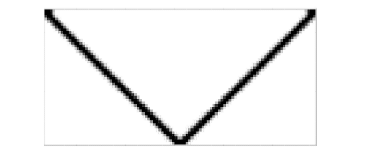Fig. 6   Optimal topology obtained by the topology optimization without fail-safe with the same displacement constraint of Fig.5(b)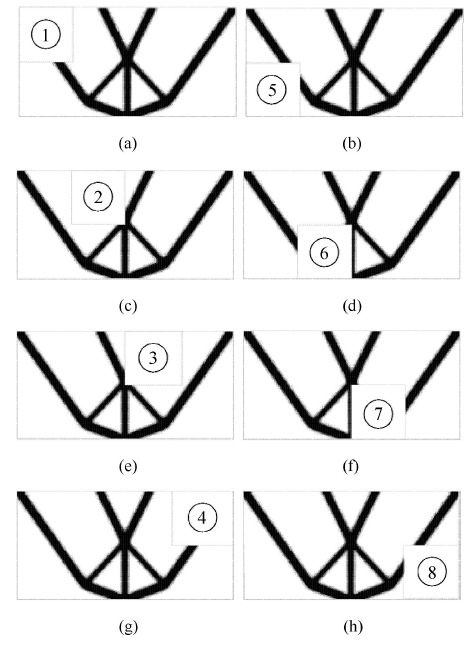Fig. 7   Topologies with different positions of fail regions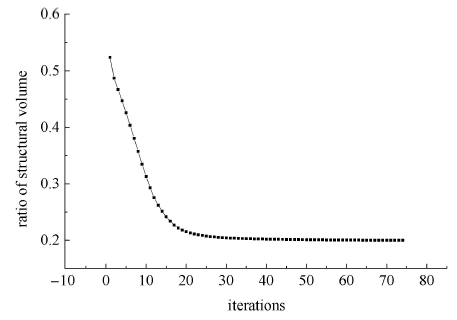Fig. 8   Iteration curve of the objective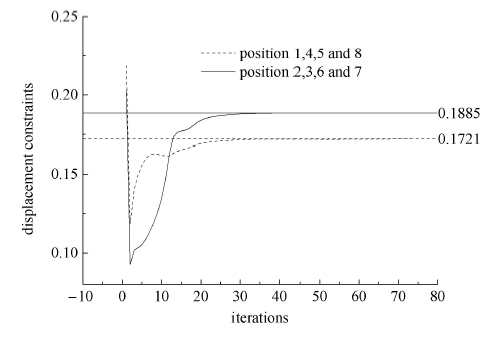Fig. 9   Iteration curves of the displacement constraints

### 3.2 算例2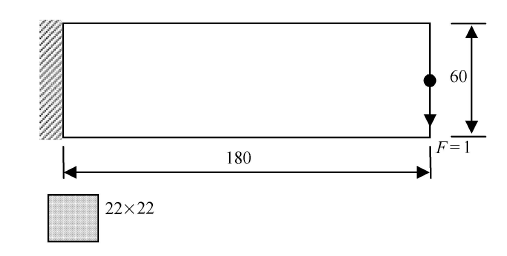Fig. 10   Definition of the topology design problem for example 2Fig. 11   Arrangement pattern of fail regions adopted in Ref. and this paper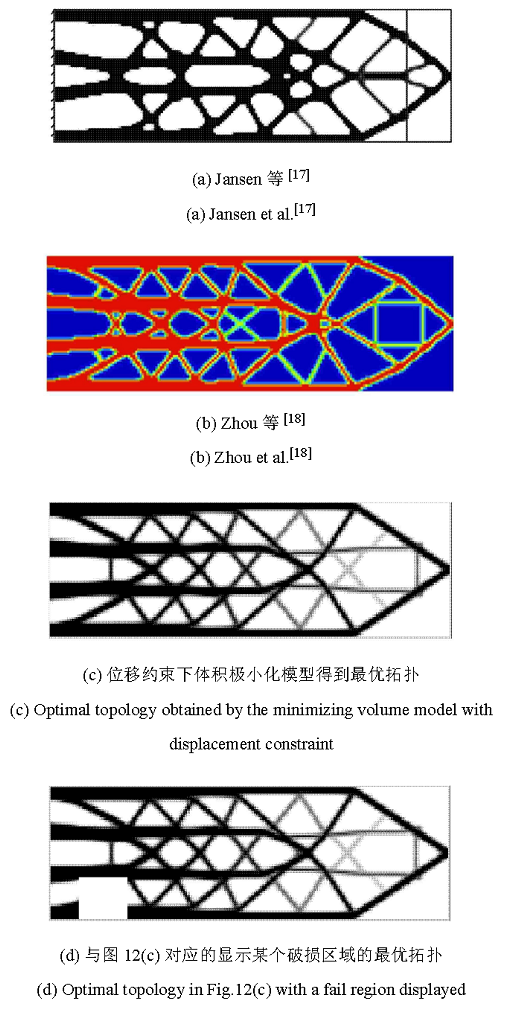Fig. 12   Optimal topologies obtained by the topology optimization with fail-safe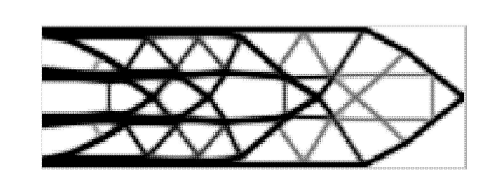Fig. 13   A transitional topology obtained by the minimizing volume model with displacement constraint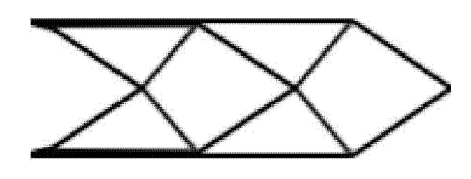Fig. 14   Optimal topologies obtained by the topology optimization without fail-safe

### 3.3 算例3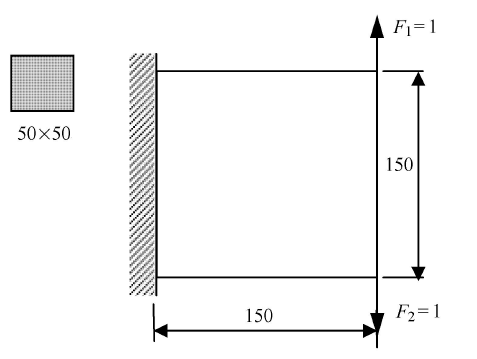Fig. 15   Definition of the topology optimization problem of a square plate with 150 ×150 mesh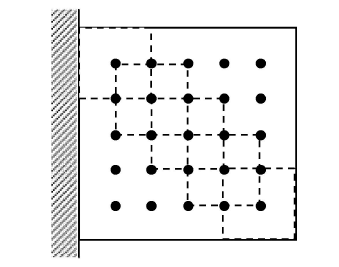Fig. 16   Arrangement of failure regions with the space of $ĕ$ in the ground structure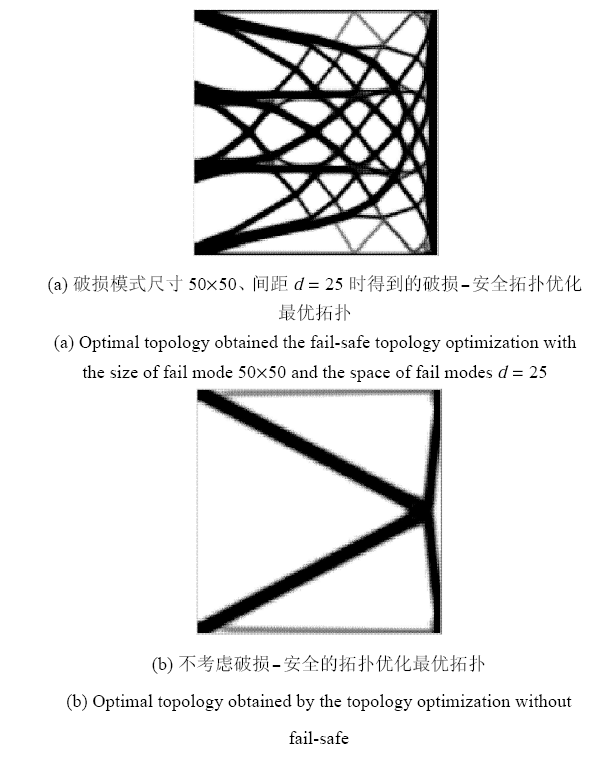Fig. 17   Optimal topologies

## 4 结 论

(1) 通过指定一个特定局部破损区域的方式模拟结构局部破损,使之破损模式确定,从而降低了连续体结构破损-安全拓扑优化问题最不利结构破损状况“双盲”困难的难度,有利于建立破损-安全拓扑优化的模型,原因在于此做法与许多工程实践相符合,如爆炸、失火、碰撞等事故通常也是发生在结构的一处局部位置.

(2)基于ICM方法建立的性能(如位移)约束下体积极小化的优化模型,不需要先验指定体积约束值,对多工况问题,也不需要人为指定工况间的加权系数组合成单目标. 取不含破损区域的基结构对应的体积为目标函数,将极小化极大值问题转化为了单目标问题. 类比于载荷工况,将在不同位置包含结构局部破损模式的基结构定义为结构破损状况,在优化模型中以约束的形式考虑,使所有结构破损状况的性能得到满足.

(3)考虑破损-安全的拓扑优化得到的最优结构,最终的结构拓扑构形更复杂,使结构具有更多的冗余,最优结构的体积也更大,亦即使用的材料更多,从而满足破损-安全设计原则的要求,以适度增加的材料用量换来结构的冗余度的增加,抵抗因偶然因素造成的结构局部破坏可能造成的灾难性后果,因此可以在预估的所有结构破损状况下满足结构的性能要求.

(4)破损模式无缝平铺于基结构或破损模式重叠排列于基结构的两种方案均可采用,不同结构破损状况的预估分布,对最优结构拓扑有显著影响,需根据结构工程应用实际情况,恰当定义结构破损状况及其预估分布. 而其对最优结构拓扑的影响是一个值得深入研究的内容.

(5)由于ICM方法与SIMP方法很多共性,我们相信在SIMP方法的框架下,建立体积极小化目标受位移约束的模型,同样可以建立类似的合理模型.

The authors have declared that no competing interests exist.

## 参考文献 原文顺序 文献年度倒序 文中引用次数倒序 被引期刊影响因子

  Niu MCY. Airframe Structural Design.Hongkong: Conmilit Press Ltd., 1988  Niu MCY.Airframe Stress Analysis and Sizing. Hongkong: Conmilit Press Ltd., 1997  Zdeněk P Bažant, Yong Z. Why does the world trade center collapse? — Simple analysis.International Journal of Structural Stability and Dynamics, 2012, 1(4): 603-615  Melosh RJ, Johnson JR, Luik R.Structural survivability analysis//Second Conference on Matrix Methods in Structural-Mechanics, WPAFB, Ohio, 1968  Bendsøe MP, Sigmund O. Topology Optimization - Theory, Methods and Applications. Berlin: Springer, 2004  Deaton J, Grandhi RV.A survey of structural and multidisciplinary continuum topology optimization: Post 2000.Struct Multidiscip Optim, 2014, 49(1): 1-38  Sun PF, Arora JS, Haug EJ.Fail-safe optimal design of structure.Engineering Optimization, 1976, 2(1): 43-53  Arora J, Govil A, Haskell D.Optimal design of large structures for damage tolerance.AIAA Journal, 1980, 18(5): 563-570  Nguyen DT, Arora JS.Fail-safe optimal design of complex structures with substructures.Journal of Mechanical Design, 1982, 104(4): 861-868  Feng Y.The theory of structural redundancy and its effect on structural design.Computers & Structures, 1988, 28(1): 15-24  Feng Y, Moses F.Optimum design, redundancy and reliability of structural systems.Computers & Structures, 1986, 24(2): 239-251  Marhadi K, Venkataraman S, Wong S.Load redistribution mechanism in damage tolerant and redundant truss structure.Structural and Multidisciplinary Optimization, 2011, 44(2): 213-233  Marhadi K, Venkataraman S.Surrogate measures to optimize structures for robust and predictable progressive failure.Structural and Multidisciplinary Optimization, 2009, 39(3): 245-261  杜剑明,郭旭. 基于鲁棒性优化的桁架结构失效-安全设计. 力学学报, 2011, 43(4): 725-730 (Du Jianming, Guo Xu.Fail-safe optimal design of truss structures based on robust optimization.Chinese Journal of Theoretical and Applied Mechanics, 2011, 43(4): 725-730 (in Chinese))  Bendsøe M, D'${\imath}$az A. A method for treating damage related criteria in optimal topology design of continuum structures.Structural and Multidisciplinary Optimization, 1998, 16(2-3): 108-115  Achtziger W, Bendsøe M.Optimal topology design of discrete structures resisting degradation effects.Structural and Multidisciplinary Optimization, 1999, 17(1): 74-78  Jansen M, Lombaert G, Schevenels M, et al.Topology optimization of fail-safe structures using a simplified local damage model.Structural and Multidisciplinary Optimization, 2013, 49(4): 657-666  Zhou M, Fleury R.Fail-safe topology optimization.Structural and Multidisciplinary Optimization, 2016, 54(7): 1225-1243  Olhoff N.Multicriterion structural optimization via bound formulation and mathematical programming.Struct Optim, 1989, 1: 11-17  彭细荣. 结构刚度优化问题模型比较研究. 湖南城市学院学报(自然科学版), 2016, 25(1): 1-4 (Peng Xirong.A comparative study on models of structural stiffness optimization problems.Journal of Hunan City University (Natural Science), 2016, 25(1): 1-4 (in Chinese))  彭细荣, 隋允康. 对连续体结构拓扑优化合理模型的再探. 固体力学学报, 2016, 37(2): 181-191 (Peng Xirong, Sui Yunkang.Further discussion on rational topology optimization model of continuum structures.Chinese Journal of Solid Mechanics, 2016, 37(2): 181-191 (in Chinese))  隋允康, 彭细荣. 结构拓扑优化ICM方法的改善. 力学学报, 2005, 37(2): 190-198 (Sui Yunkang, Peng Xirong. The improvement for the ICM method of structural topology optimization. Acta Mechanica Sinica, 2005, 37(2): 190-198(in Chinese))  隋允康, 叶红玲. 连续体结构拓扑优化的ICM方法. 北京: 科学出版社, 2013 (Sui Yunkang, Ye Hongling.Continuum Topology Optimization Methods ICM. Beijing: Science Press, 2013 (in Chinese))  隋允康, 彭细荣.求解一类可分离凸规划的对偶显式模型DP-EM方法. 力学学报,2017, 49(5): 1135-1143 (Sui Yunkang, Peng Xirong.A dual explicit model based DP-EM method for solving a class separable convex programming.Chinese Journal of Theoretical and Applied Mechanics, 2017, 49(5): 1135-1143 (in Chinese))  Andreassen E, Clausen A, Lazarov BS, et al.Efficient topology optimization in MATLAB using 88 lines of code.Structural Multidisciplinary Optimization, 2011, 43(1): 1-16/

 〈〉